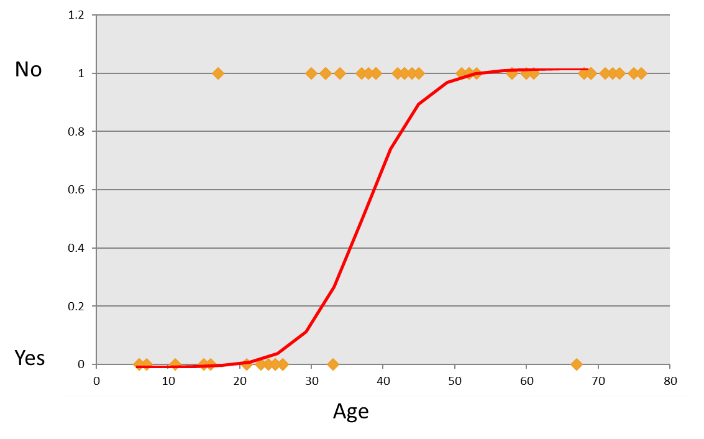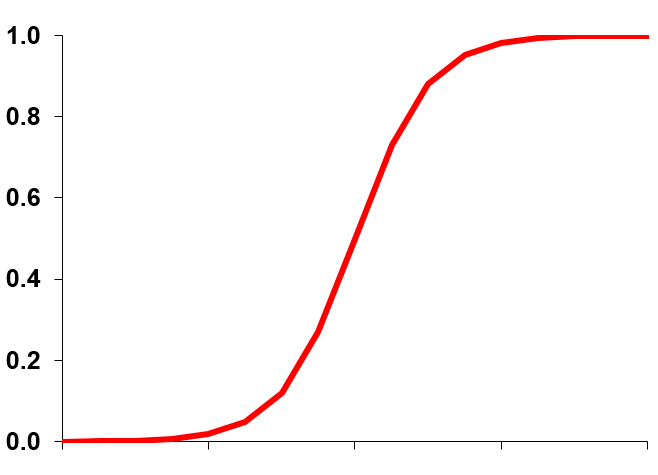• No products in the cart.

# 204.2.2 Logistic Function to Regression

##### From function to regression.

Link to the previous post :  https://statinfer.com/204-2-1-logistic-regression-why-do-we-need-it/

In the last post we saw linear regression cannot be used if the final output is binary, yes or no. As it’s tough to fit a binary output on a linear function.

To solve this problem we can move toward some different kind of functions, a Logistic Function being the first choice.

### A Logistic Function

This is how a Logistic Function look like:### The Logistic function

• We want a model that predicts probabilities between 0 and 1, that is, S-shaped.
• There are lots of s-shaped curves. We use the logistic model:
Probability=e(β0+β1X)1+e(\$β0+β1X)### Logistic Regression Output

• In logistic regression, we try to predict the probability instead of direct values.
• Y is binary, it takes only two values 1 and 0 instead of predicting 1 or 0 we predict the probability of 1 and probability of zero.
• This suits aptly for the binary categorical outputs like YES vs NO; WIN vs LOSS; Fraud vs Non Fraud.

### Practice : Logistic Regression

• Dataset: Product Sales Data/Product_sales.csv
• Build a logistic Regression line between Age and buying
• A 4 years old customer, will he buy the product?
• If Age is 105 then will that customer buy the product?
In :
import pandas as pd

import statsmodels.formula.api as sm

# Build a logistic Regression line between Age and buying
logit=sm.Logit(sales['Bought'],sales['Age'])
logit

Out:
<statsmodels.discrete.discrete_model.Logit at 0x203ba4ac630>
In :
result = logit.fit()
result

Optimization terminated successfully.
Current function value: 0.584320
Iterations 5

Out:
<statsmodels.discrete.discrete_model.BinaryResultsWrapper at 0x203bbd90e48>
In :
result.summary()

Out:
Dep. Variable: No. Observations: Bought 467 Logit 466 MLE 0 Sun, 16 Oct 2016 0.1478 14:35:42 -272.88 True -320.21 nan
coef std err z P>|z| [95.0% Conf. Int.] 0.0294 0.003 8.813 0.000 0.023 0.036
In :
###coefficients Interval of each coefficient

print (result.conf_int())

            0         1
Age  0.022851  0.035923

In :
#One more way of fitting the model
from sklearn.linear_model import LogisticRegression
logistic = LogisticRegression()
logistic.fit(sales[["Age"]],sales["Bought"])

Out:
LogisticRegression(C=1.0, class_weight=None, dual=False, fit_intercept=True,
intercept_scaling=1, max_iter=100, multi_class='ovr', n_jobs=1,
penalty='l2', random_state=None, solver='liblinear', tol=0.0001,
verbose=0, warm_start=False)
In :
#A 4 years old customer, will he buy the product?
age1=4
predict_age1=logistic.predict(age1)
print(predict_age1)



In :
#If Age is 105 then will that customer buy the product?
age2=105
predict_age2=logistic.predict(age2)
print(predict_age2)



The next post is on multiple logistic regression.
Link to the next post: https://statinfer.com/204-2-3-multiple-logistic-regression/


21st June 2017
0 responses on "204.2.2 Logistic Function to Regression"